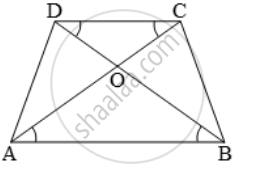# In a trapezium ABCD, O is the point of intersection of AC and BD, AB || CD and AB = 2 × CD. If the area of ∆AOB = 84 cm^2 . Find the area of ∆COD - Mathematics

Sum

In a trapezium ABCD, O is the point of intersection of AC and BD, AB || CD and AB = 2 × CD. If the area of ∆AOB = 84 cm2 . Find the area of ∆COD

#### Solution

In ∆AOB and ∆COD, we have∠OAB = ∠OCD (alt. int. ∠s)

∠OBA = ∠ODC (alt. int. ∠s)

∴ ∆AOB ~ ∆COD [By AA-similarity]

\Rightarrow \frac{ar\ (\Delta AOB)}{ar\ (\Delta COD)}=(AB^2)/(CD^2)=(2CD)^2/(CD^2) [∵ AB = 2 × CD]

=>(4xxCD^2)/(CD^2)=4

⇒ ar (∆COD) = 1/4 × ar (∆AOB)

=>(1/4xx84)cm^2=21cm^2

Hence, the area of ∆COD is 21 cm2 .

Concept: Areas of Similar Triangles
Is there an error in this question or solution?